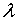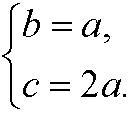7. Lagrange multipliers.

In the figure below we have illustrated an extreme value problem with constraints. The point A is the largest value of the function z=f(x,y) while the point B is the largest value of the function under the constraintg(x,y)=0.Theorem: (Lagrange multipliers) Let f and g be differntiable functions, where f’x(x0,y0) and f’y(x0,y0) not both are zero. If the function f(x,y) has an extreme value in the point (x0,y0) under the constraint g(x,y)=0, then there is a constantsuch thatwhereRemark: A similar theorem holds for a general function f(x1,x2,…,xn) with m constraints.

Example: The case n=3, m=2. Write the constraints as g1(x,y,z)=0 and g2(x,y,z)=0 and considerAn (if existent) extreme value can be found by solving the systemExample 6: How to construct a box with the same shape as in the figure below with a fix volume V0 such that the amount of material used will be minimal?Solution: The problem consists of minimizing the amount of material (proportional to the area)under the constraintBy Lagrange’s multiplier method we therefore constructand then solve the systemMultiply the first equation with a, the second with b and the third with c. By using that abc=V0, we getIf we subtract the second equation from the first we getsince a,b,c>0. If we then put a=b in the last two equations we getNow subtract the second of these two equations from the firstWe should thus chooseInserted in the expression for the volumen we then haveThis imples that we should choose